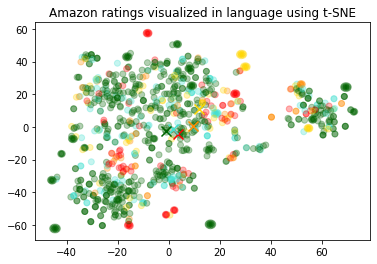# Visualizing the embeddings in 2D

,
Mar 10, 2022
Open in Github

We will use t-SNE to reduce the dimensionality of the embeddings from 1536 to 2. Once the embeddings are reduced to two dimensions, we can plot them in a 2D scatter plot. The dataset is created in the Get_embeddings_from_dataset Notebook.

### 1. Reduce dimensionality

We reduce the dimensionality to 2 dimensions using t-SNE decomposition.

``````import pandas as pd
from sklearn.manifold import TSNE
import numpy as np
from ast import literal_eval

datafile_path = "data/fine_food_reviews_with_embeddings_1k.csv"

# Convert to a list of lists of floats
matrix = np.array(df.embedding.apply(literal_eval).to_list())

# Create a t-SNE model and transform the data
tsne = TSNE(n_components=2, perplexity=15, random_state=42, init='random', learning_rate=200)
vis_dims = tsne.fit_transform(matrix)
vis_dims.shape``````
`(1000, 2)`

### 2. Plotting the embeddings

We colour each review by its star rating, ranging from red to green.

We can observe a decent data separation even in the reduced 2 dimensions.

``````import matplotlib.pyplot as plt
import matplotlib
import numpy as np

colors = ["red", "darkorange", "gold", "turquoise", "darkgreen"]
x = [x for x,y in vis_dims]
y = [y for x,y in vis_dims]
color_indices = df.Score.values - 1

colormap = matplotlib.colors.ListedColormap(colors)
plt.scatter(x, y, c=color_indices, cmap=colormap, alpha=0.3)
for score in [0,1,2,3,4]:
avg_x = np.array(x)[df.Score-1==score].mean()
avg_y = np.array(y)[df.Score-1==score].mean()
color = colors[score]
plt.scatter(avg_x, avg_y, marker='x', color=color, s=100)

plt.title("Amazon ratings visualized in language using t-SNE")``````
`Text(0.5, 1.0, 'Amazon ratings visualized in language using t-SNE')`